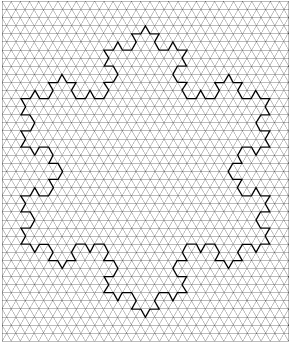## Answers for Chapter 4 Sample Problems

Snowflake curve #4:The areas of the snowflake curves:
• A1 = 1
• A2 = 1 + 3/9
• A3 = 1 + 3/9 + 12/81
• A4 = 1 + 3/9 + 12/81 +
• A = 1 + 3/9 + 12/81 + ...

The perimeters of the snowflake curves:
• P1 = 1
• P2 = 1 + 3/9
• P3 = 1 + 3/9 + 4/9
• P4 =
• P = 1 + 3/9 + 4/9 + ...
If you are interested, and you've worked hard to find the area and perimeter, then go to Emily's work.
Go back to problems from Ch. 4